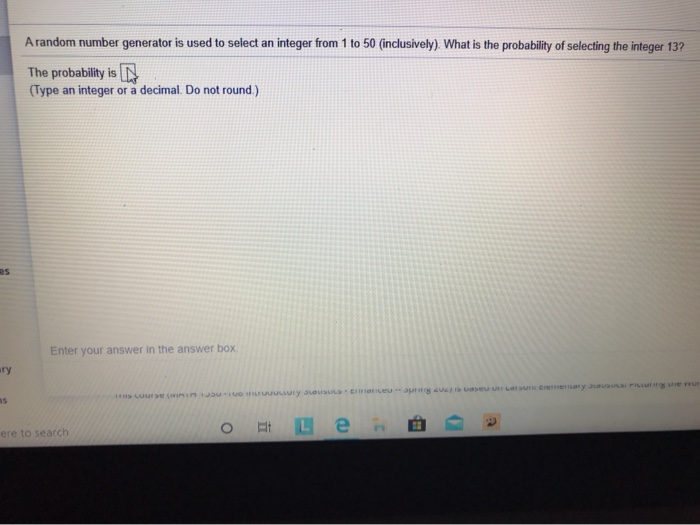# Random number generator 1-50A random number generator is used to select an integer from 1 to 50 (inclusively). What is the probability of selecting the integer 13? The probability is N (Type an integer or a decimal. Do not round) Enter your answer in the answer box ary emo ULLY D U LUI OLED Cry D o Le @ ere to search PPSS Nineteen of the 100 digital video recorders (DVRs) in an inventory are known to be defective. What is the probability you randomly select a DVR that is not defective? The probability is (Type an integer or a decimal. Do not round.) Next Question Enter your answer in the answer box Tary ons o Le here to search PSD

1) A random number generator is used to select an integer from 1
to 50. Since random number generator generates any number randomly,
all the numbers from 1 to 50 get equal chance to occur.
Thus if X denote the random number selected by using the random
number generator, then,
P(X=k) =1/50, k=1, 2,…,50,i.e., X follows a discrete uniform
distribution.
Thus, P(X=13) =1/50=0.02.
Thus, the required probability is 0.02.
2) Given, there are 19 DVRs among 100 DVRs which are known to be
defective.
Thus, there are 81 non defective DVRs and any one of them can be
chosen in 81C1=81 possible ways.
Again any one DVR from 100 DVR can be chosen in
100C1=100 possible ways.
Thus, the probability of randomly selecting a non defective DVR
is, 81/100=0.81.
Thus the required probability is 0.81.